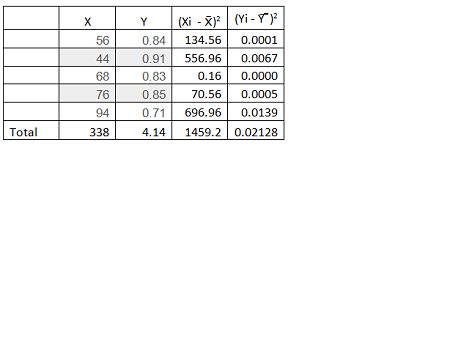# Given the data as shown in the table below Assuming the correlation coefficient of X and Y is...

## Question:

Given the data as shown in the table below Assuming the correlation coefficient of X and Y is found to be -0.897995, calculate the slope, m, and y-intercept, b, of the "Best-Fit" line Y = mX + b. Use 4 decimal places for each of x, y values obtained from your calculation. Round your answer to 4 decimal places. Using the equation of the "Best-Fit" line to predict the value of Y when X = 60. Enter your answer in 4 decimal places. Using the equation of the "Best-Fit" line to predict the value of X and Y = 0.89. Enter your answer in 4 decimal places.

 X Y 56 0.84 44 0.91 68 0.83 76 0.85 94 0.71

## Regression

Regression determines the strength of relationship between the dependent variables. There are many types of regression which include Linear regression, Polynomial regression, Logistic regression, etc.

It is mostly used in finance and investing fields.

## Answer and Explanation: 1

Become a Study.com member to unlock this answer! Create your account{eq}\begin{align*} \overline X \; &= \;\dfrac{{\sum X }}{n}\\ & = \dfrac{{338}}{5}\\ & = 67.6 \end{align*} {/eq}

{eq}\begin{align*} \overl...

See full answer below.

#### Learn more about this topic:Simple Linear Regression: Definition, Formula & Examples

from

Chapter 8 / Lesson 2
32K

Simple linear regression is a great way to make observations and interpret data. In this lesson, you will learn to find the regression line of a set of data using a ruler and a graphing calculator.